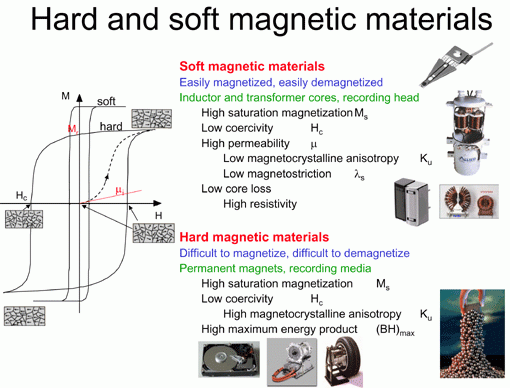MR Sensor Technology

Magnetic Sensor Materials

Date：03-12-2015    Hits：9989Magnetic materials – based magnetic field sensors are used in a wide range of applications in the arketplace. Experiments show that a given material, placed in a magnetic field to be magnetized, is magnetizable to varying degrees. Materials can be divided into five categories according to the response of their magnetization to an applied magnetic field: diamagnetic, paramagnetic, ferromagnetic, ferrimagnetic, and antiferromagnetic.

(1) Diamagnetic material has a relative magnetic susceptibility ( r) < 1. It has a complete outer electron atomic shell, so it has no magnetic moment. However, an applied magnetic field will produce electron rotation (Larmor precession).This precession in the magnetic field is in a direction opposite to the applied field. Thus, the induced magnetization is opposite the applied field. The diamagnetic susceptibility is independent of temperature.

(2) Paramagnetic material has a relative magnetic susceptibility  r > 1. This means that the magnetization of a paramagnetic material is parallel to the direction of the applied magnetic field.  For both paramagnetic and diamagnetic materials, the net magnetization is linearly proportional to the applied field, and usually not much different than the magnetization of vacuum ( r = 1).

(3) Ferromagnetic material has mr >> 1.  Its net magnetization responds very strongly and parallel to an applied magnetic field.  Small regions called “magnetic domains” form within a sample of ferromagnetic materials.  Within the volume of a magnetic domain, the magnetization is in a uniform direction.  The magnetization of adjacent domains may be at different, and random, directions.  The net magnetization of a sample is the sum of the magnetization of each magnetic domain.  This value can be zero if the domain magnetization directions are randomized. But when a large enough magnetic field is applied, all of the magnetic domains are pointing parallel to the applied field and the net magnetization is very large.

For the transition metal elements (iron, nickel, and cobalt) the atomic magnetic moment comes from an unpaired electron spin.  In the rare earth elements, the electron orbit imbalances also contribute to the magnetic moment.  At the magnetic domain level, there are two kinds of change processes that occur: re-orientation within a domain, and

growing or shrinking of a given domain. For small applied fields, these magnetization changes can be “reversible” in a conservation of energy sense.

The magnetic susceptibility of ferromagnetic materials decreases with temperature up to the point of dipping rapidly towards 1. This temperature is called the Curie point, which is different for each element.  Some Curie points are: iron 730°, cobalt 1131°, and nickel 358°.  This effect is due to the thermal energy vibrating the magnetic moments and tending to randomize their magnetizations.  Above the Curie point, the material is paramagnetic.

(4) Ferrimagnetic material has an internal crystal structure that is a lattice of alternatively oriented magnetic moments.  Some are pointed parallel to the applied field, others anti-parallel.  But because the magnitude of the anti-parallel moments is smaller than the parallel ones, there is a net magnetic moment (non-zero).

Ferrimagnetic materials do have magnetic domains and a Curie point, but the temperature dependence is very complex.  They generally have a high electrical resistivity. Examples include magnetic iron oxide with one or more other metal oxide (manganese, nickel, or zinc), and are referred to as “ferrites”.

(5)  Anti-ferromagnetic materials are similar to Ferrimagnetic in that there is a lattice with two opposite directions of magnetic moment.  But in an antiferromagnetic material, the magnitude of the opposing moments is equal, so they very nearly cancel each other in the total net magnetic moment.

These magnetic material effects can be used to measure the internal magnetic properties of a device.  This is because some of their physical properties change, such as the resistance as a function of magnetization direction.

Next: Spintronics

•